### Home > CALC > Chapter 2 > Lesson 2.3.2 > Problem2-119

2-119.
1.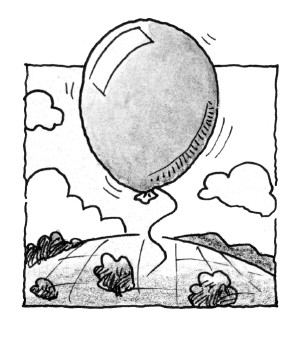When the balloon in problem 2-81 reached 500 feet, it popped and started to fall back towards the ground. The height of the balloon as it falls is modeled by the function h(t) = −16t2 + 500, where t is the number of seconds since the time it popped and h(t) is the height of the balloon (in feet) above the ground. Homework Help ✎

1. According to h(t), when will the balloon hit the ground?

2. Approximate the balloon's velocity at t = 5 seconds.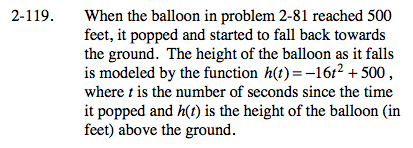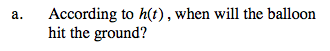What value of h represents the ground?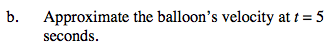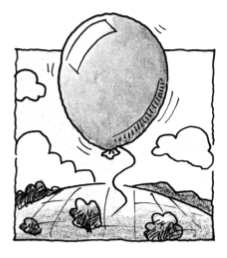Velocity is slope of a distance/position graph. Since h(t) is a parabola, we do not have the skills (yet) to find the slope on a curve. But we can approximate.

Choose two times very close to t = 5. Use the function, h(t), to find the balloon's height at those times. Find the slope of the line that goes through those points. This will be a good approximation of the velocity of the balloon at t = 5.